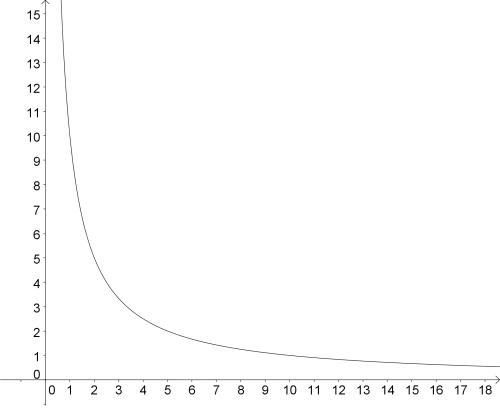#### You may also like### Real(ly) Numbers

If x, y and z are real numbers such that: x + y + z = 5 and xy + yz + zx = 3. What is the largest value that any of the numbers can have?### Overturning Fracsum

Can you solve the system of equations to find the values of x, y and z?### Pair Squares

The sum of any two of the numbers 2, 34 and 47 is a perfect square. Choose three square numbers and find sets of three integers with this property. Generalise to four integers.

# Graphs of Changing Areas

##### Age 16 to 18Challenge Level

In the problem Changing Areas, Changing Perimeters, you are invited to arrange some rectangles according to their area and perimeter. This problem invites you to consider properties of graphs related to areas and perimeters of rectangles.

The graph below shows the curve $y=\frac{10}{x}$.Imagine $x$ and $y$ are the length and width of a rectangle.
Each point on the curve represents a rectangle - what property do these rectangles share?

What symmetry does the graph have? How do you know?
What happens to the graph as $x$ gets very large? How do you know?

You could plot graphs of other curves such as $y=\frac{5}{x}$ or $y=\frac{20}{x}$.
How would these graphs relate to the one above? Would the graphs intersect? How do you know?

Rectangles of equal perimeter can be represented graphically by the line $y=\frac{1}{2}P-x$ where $P$ is the perimeter.
Would you expect the line $y=\frac{1}{2}P-x$ to intersect with the curve $y=\frac{10}{x}$ for all values of $P$?

How can you use the graph to find the smallest possible perimeter of a rectangle with an area of $10$?